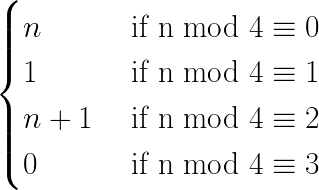# Mysterious Pattern with Exclusive OR (XOR) Sum.

I am going to tell a story about a pattern that i found while i was having lunch. Last Friday, i was presented with a problem which its solution is an xor sum of a table that contains consecutive numbers starting from zero.XOR Gate

Well back to the lunch timeline, i got an idea of grabbing a paper and start simulating the sum, but this time using the binary form. So i did, the first seven numbers didn’t look similar, but i wanted to continue until i reached eleven. What i noticed is that in fact there is a pattern. Mysteriously, every four numbers resets the sum to zero and the values are accurately predictable.

Lets suppose that we have a number n that we want to xor it with the previous sum (n-1 xor n-2….xor 0) Let’s have a small simulation:

0 xor 0= 0 / n = 0, result=n

1 xor 0 = 1 / n=1, result=1

2 xor 1 = 3 / n=2, result=n+1

3 xor 3 = 0 / n=3, result = 0

4 xor 0) = 4 / n=4, result=n

5 xor 4= 1 / n=5, result=1

6 xor 1 = 7 / n=6, result=n+1

7 xor 7 = 0 / n=7, result=0

8 xor 0 = 8 / n=8, result=n

9 xor 8 = 1 / n=9, result=1

10 xor 1 = 11 / n=10, result=n+1

11 xor 11 = 0 / n=11, result=0

12 xor 0 = 12 / n=12, result=n

One pattern is noticeable here is “every four numbers resets the sum to zero, with that in mind, the next counter can be predicted to be (n, 1, n+1 or 0).

Analyzing the pattern, well for every four number the pattern is reset, let’s just write it in a formula and try to determine a pattern.

first let’s start with the second cycle:

• For the ones that returns n we have: 4,8,12, 16….4*k where k in N.

I didn’t believe it at first, so i ran a simulation verifying this property from 0 to 2⁶⁴ and it work and continued to work.

If i can generalize it in a formula, it would be like this:Generalized XOR sum formula

Using this formula we can determine any xor sum, for example:

XorSum(0..99)= 0 because 99 % 4=3

XorSum(0..200)= 200 because 200 % 4=0

Well i don’t know if there is a mathematical theorem for that, i just wanted to share it with people, so if someone knows it, please let me know so i can learn the name. I hope it helps anyone in need.

We new things everyday and lets continue to do that together.

.NET Developer/Junior DevOps/Junior CTO/Experienced C# developer (https://www.linkedin.com/in/arsslen-idadi)

## More from Arsslen Idadi

.NET Developer/Junior DevOps/Junior CTO/Experienced C# developer (https://www.linkedin.com/in/arsslen-idadi)